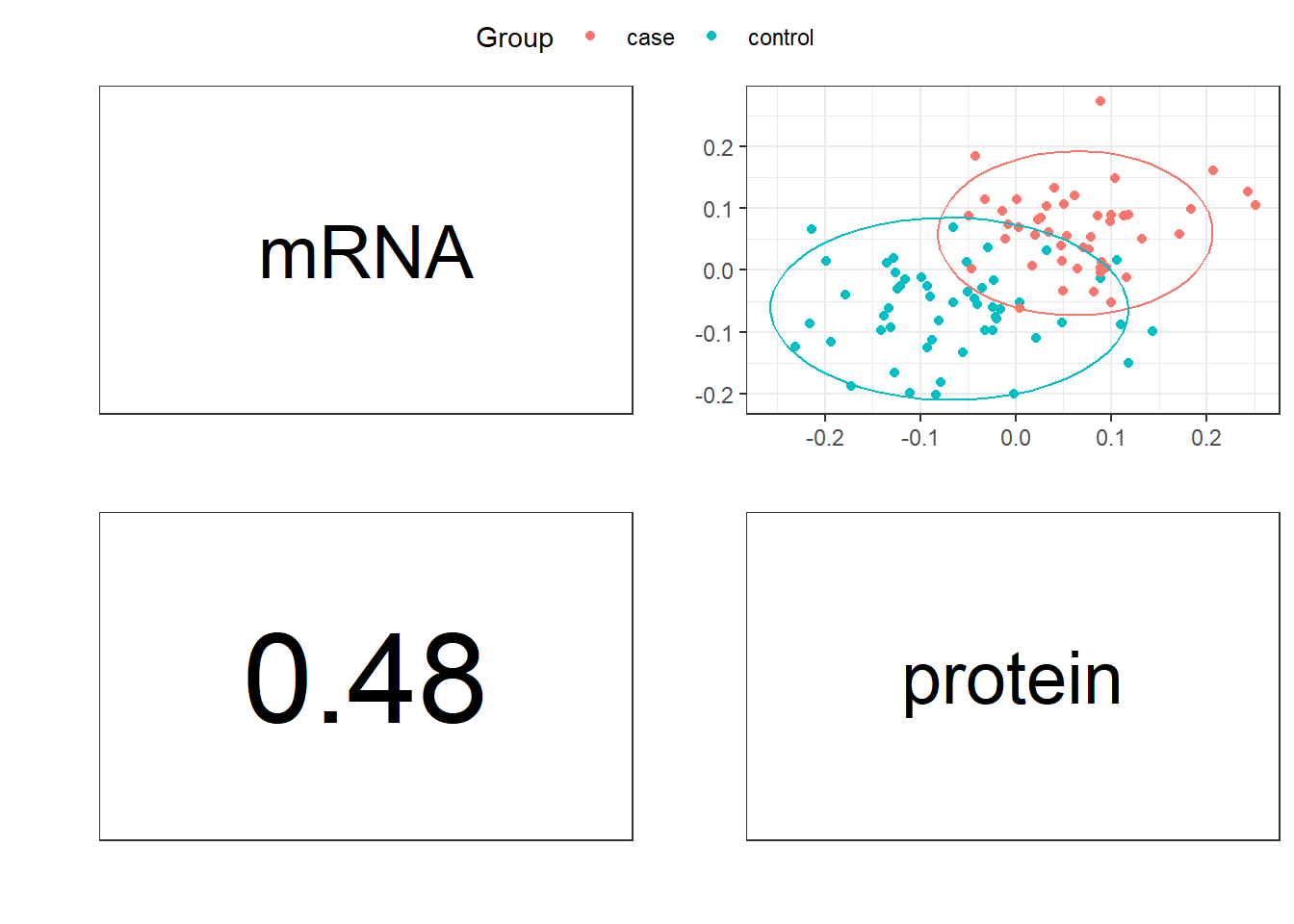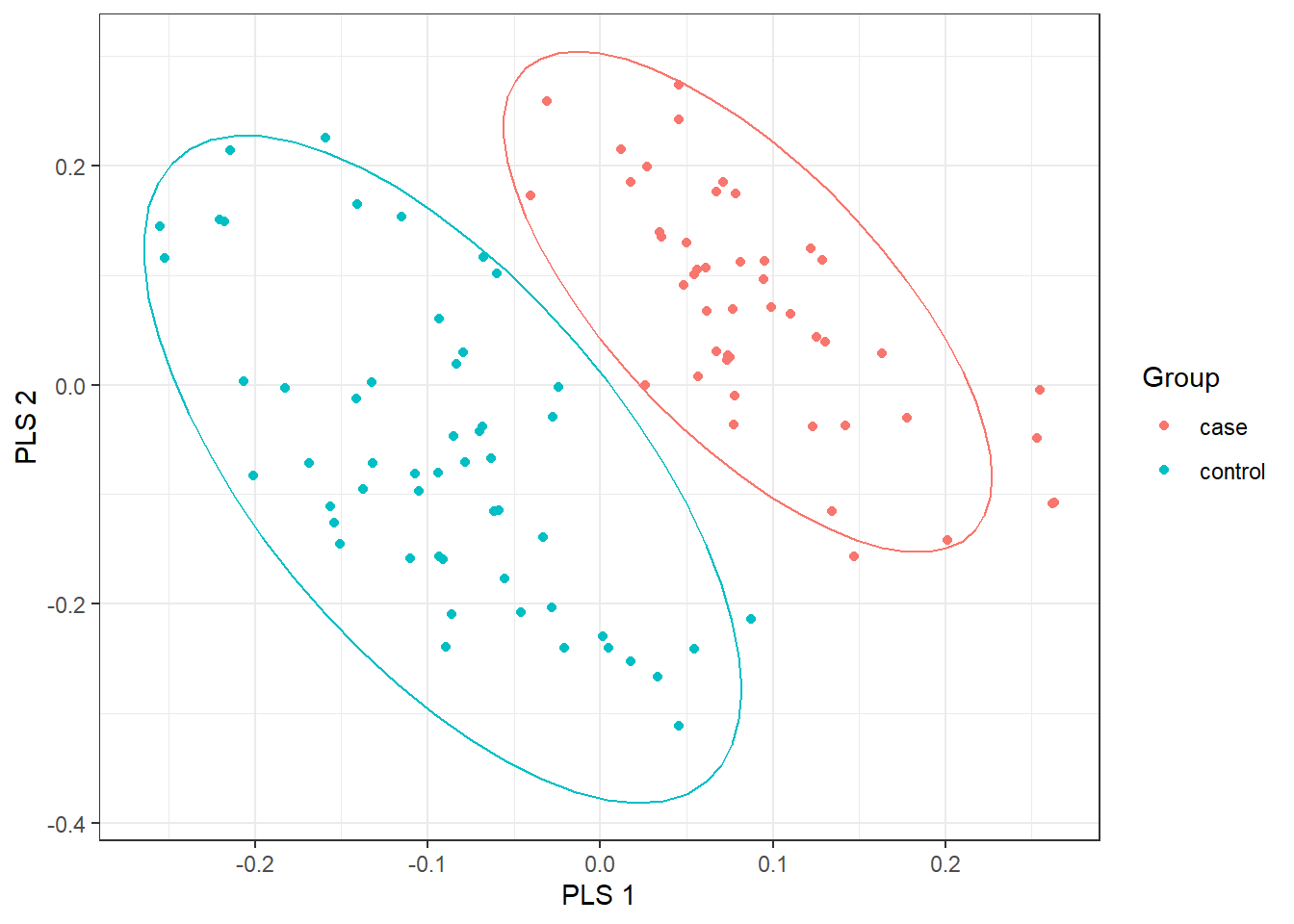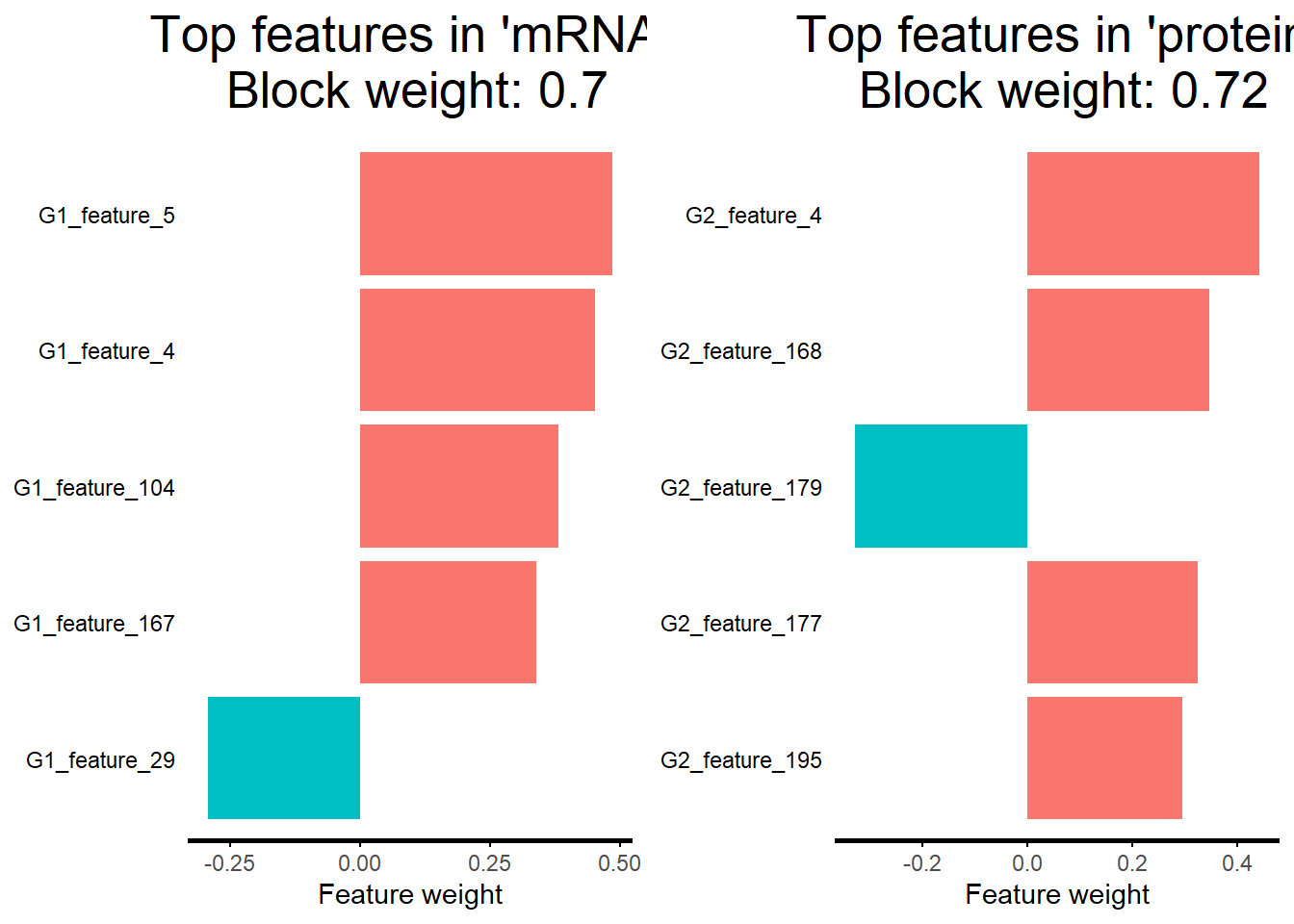# 1 Requirements

Please install the R package prior to use asmbPLS.

library(asmbPLS) ## load the R package
set.seed(123) ## set seed to generate the same results

If you want to see the list of functions in asmbPLS, please use ?asmbPLS.
If you want to see the detailed description for each function, please use ?function, for example ?asmbPLS.cv.

# 2 asmbPLS

## 2.1 Example data for asmbPLS algorithm

data(asmbPLS.example) ## load the example data set for asmbPLS

8 components are included in the asmbPLS.example:
1) X.matrix, a matrix with 100 samples (rows) and 400 features (columns, 1-200 are microbial taxa, 201-400 are metabolites);
2) X.matrix.new, a matrix to be predicted with 100 samples (rows) and 400 features (columns, 1-200 are microbial taxa, 201-400 are metabolites);
3) Y.matrix, a matrix with 100 samples (rows) and 1 column (log-transformed survival time);
4) X.dim, dimension of the two blocks in X.matrix;
5) PLS.comp, selected number of PLS components;
6) quantile.comb, selected quantile combinations;
7) quantile.comb.table.cv, pre-defined quantile combinations for cross validation;
8) Y.indicator, a vector containing the event indicator for each sample.

Pre-processing has been applied to the two different types of data in X.matrix.
Different types of omics data require specific pre-processing steps tailored to their unique characteristics.

## show the first 5 microbial taxa and the first 5 metabolites for the first 5 samples.
asmbPLS.example$X.matrix[1:5, c(1:5, 201:205)] #> Taxa_1 Taxa_2 Taxa_3 Taxa_4 Taxa_5 metabolite_1 #> [1,] -1.63175165 2.4625929 2.6119703 -0.7154609 -4.1166583 6.900372 #> [2,] -3.27012529 1.9821481 -0.3256863 0.1956106 0.6617003 8.074607 #> [3,] 1.81855927 -0.5863047 1.7614009 -2.0526417 -3.8444012 7.669377 #> [4,] -4.29551101 -0.8943136 0.3488799 1.0889840 2.5439654 7.876529 #> [5,] 0.03458501 0.9299691 -0.4423391 2.7631137 -4.0258580 6.538369 #> metabolite_2 metabolite_3 metabolite_4 metabolite_5 #> [1,] 3.905864 3.411215 5.802873 5.910157 #> [2,] 2.919310 5.032784 6.013281 5.080354 #> [3,] 3.815648 6.653860 4.064987 5.377000 #> [4,] 1.731010 4.232027 6.144947 5.382338 #> [5,] 3.829668 4.680280 4.678092 5.505065 ## show the outcome for the first 5 samples. asmbPLS.example$Y.matrix[1:5,]
#>  6.410580 5.899169 5.684940 7.034513 5.842238

## 2.2 Cross Validation

The 5-fold CV with 5 repetitions is implemented to help find the best quantile combination for each PLS component as well as the optimal number of PLS components.

X.matrix = asmbPLS.example$X.matrix X.matrix.new = asmbPLS.example$X.matrix.new
Y.matrix = asmbPLS.example$Y.matrix PLS.comp = asmbPLS.example$PLS.comp
X.dim = asmbPLS.example$X.dim quantile.comb.table.cv = asmbPLS.example$quantile.comb.table.cv
Y.indicator = asmbPLS.example$Y.indicator ## cv to find the best quantile combinations for model fitting cv.results <- asmbPLS.cv(X.matrix = X.matrix, Y.matrix = Y.matrix, PLS.comp = PLS.comp, X.dim = X.dim, quantile.comb.table = quantile.comb.table.cv, Y.indicator = Y.indicator, k = 5, ncv = 5) ## obtain the best quantile combination for each PLS component quantile.comb <- cv.results$quantile_table_CV[,1:length(X.dim)]
## obtain the optimal number of PLS components
n.PLS <- cv.results$optimal_nPLS  ## 2.3 Model Fit The selected quantile combination for each PLS component and the optimal number of PLS components can be used as input for the asmbPLS.fit function to fit the final model. asmbPLS.results <- asmbPLS.fit(X.matrix = X.matrix, Y.matrix = Y.matrix, PLS.comp = n.PLS, X.dim = X.dim, quantile.comb = quantile.comb) ## 2.4 Prediction Once the model is fitted, you can use the model to do the prediction using the new data set (X.matrix.new). Y.pred <- asmbPLS.predict(asmbPLS.results, X.matrix.new, n.PLS) head(Y.pred$Y_pred)
#>          [,1]
#> [1,] 6.064187
#> [2,] 6.764513
#> [3,] 6.238559
#> [4,] 5.912458
#> [5,] 7.243043
#> [6,] 6.143728

Also, you can do the prediction using the original data set X.matrix to check the model fit.

## prediction for original data to check the data fit
Y.fit <- asmbPLS.predict(asmbPLS.results, X.matrix, n.PLS)
check.fit <- cbind(Y.matrix, Y.fit$Y_pred) head(check.fit) #> [,1] [,2] #> [1,] 6.410580 6.374432 #> [2,] 5.899169 6.108969 #> [3,] 5.684940 5.408893 #> [4,] 7.034513 6.961441 #> [5,] 5.842238 5.759226 #> [6,] 7.429254 6.833743 # 3 asmbPLS-DA ## 3.1 Example data for asmbPLS-DA algorithm data(asmbPLSDA.example) ## load the example data set for asmbPLS-DA 8 components are included in the asmbPLSDA.example: 1) X.matrix, a matrix with 100 samples (rows) and 400 features, features 1-200 are from block 1 and features 201-400 are from block 2; 2) X.matrix.new, a matrix to be predicted with 100 samples (rows) and 400 features, features 1-200 are from block 1 and features 201-400 are from block 2; 3) Y.matrix.binary, a matrix with 100 samples (rows) and 1 column; 4) Y.matrix.morethan2levels, a matrix with 100 samples (rows) and 3 columns (3 levels); 5) X.dim, dimension of the two blocks in X.matrix; 6) PLS.comp, selected number of PLS components; 7) quantile.comb, selected quantile combinations; 8) quantile.comb.table.cv, pre-defined quantile combinations for cross validation. ## show the first 5 features from block 1 and the first 5 features from block 2 for the first 5 samples. asmbPLSDA.example$X.matrix[1:5, c(1:5, 201:205)]
#>      G1_feature_1 G1_feature_2 G1_feature_3 G1_feature_4 G1_feature_5
#> [1,]    0.2444821    0.3715013  -0.21780418   0.58436337  -1.19637830
#> [2,]   -0.2323967   -0.2874724  -0.31100383  -0.11253287  -0.03668811
#> [3,]   -1.0820464    0.4585036   0.03601036  -0.76487964   0.72843992
#> [4,]    1.0914838    2.0047015  -0.15045351   0.16187751  -1.24755651
#> [5,]    1.0288807   -1.0768499  -0.12903603   0.08122353   1.75583752
#>      G2_feature_1 G2_feature_2 G2_feature_3 G2_feature_4 G2_feature_5
#> [1,]   -0.7820185  -0.05832018   -2.3319625   -1.6828910    0.9156531
#> [2,]   -0.4364726  -2.19203919   -0.1741337   -1.3169466    0.3992667
#> [3,]   -0.1695784   0.24391203    0.8735221    0.5688791   -0.1548541
#> [4,]    0.8182932   1.38808419   -0.9268429   -1.8371658    1.1532969
#> [5,]   -0.8297609   0.01371050    0.6736516   -0.7678065   -0.7571429
## show the binary outcome for the first 5 samples.
asmbPLSDA.example$Y.matrix.binary[1:5,] #>  0 0 1 1 1 ## show the multiclass outcome for the first 5 samples. asmbPLSDA.example$Y.matrix.morethan2levels[1:5,]
#>      [,1] [,2] [,3]
#> [1,]    0    1    0
#> [2,]    0    0    1
#> [3,]    0    1    0
#> [4,]    0    1    0
#> [5,]    0    1    0

In the example data set, we include both binary outcome and multiclass outcome.

## 3.2 Cross Validation

Similarly, the 5-fold CV with 5 repetitions is implemented to help find the best quantile combination for each PLS component as well as the optimal number of PLS components.
You can use different decision rules (method in the function, the default is fixed_cutoff for the binary outcome and Max_Y for the multiclass outcome) and different measure (The default is balanced accuracy B_accuracy) for the CV.
Also, note that you need to set different outcome.type for different types of outcomes.
Extract the components from the example data list:

X.matrix = asmbPLSDA.example$X.matrix X.matrix.new = asmbPLSDA.example$X.matrix.new
Y.matrix.binary = asmbPLSDA.example$Y.matrix.binary Y.matrix.multiclass = asmbPLSDA.example$Y.matrix.morethan2levels
X.dim = asmbPLSDA.example$X.dim PLS.comp = asmbPLSDA.example$PLS.comp
quantile.comb.table.cv = asmbPLSDA.example$quantile.comb.table.cv CV for the binary outcome: ## cv to find the best quantile combinations for model fitting (binary outcome) cv.results.binary <- asmbPLSDA.cv(X.matrix = X.matrix, Y.matrix = Y.matrix.binary, PLS.comp = PLS.comp, X.dim = X.dim, quantile.comb.table = quantile.comb.table.cv, outcome.type = "binary", k = 5, ncv = 5) quantile.comb.binary <- cv.results.binary$quantile_table_CV[,1:length(X.dim)]
n.PLS.binary <- cv.results.binary$optimal_nPLS CV for the multiclass outcome: ## cv to find the best quantile combinations for model fitting ## (categorical outcome with more than 2 levels) cv.results.multiclass <- asmbPLSDA.cv(X.matrix = X.matrix, Y.matrix = Y.matrix.multiclass, PLS.comp = PLS.comp, X.dim = X.dim, quantile.comb.table = quantile.comb.table.cv, outcome.type = "multiclass", k = 5, ncv = 5) quantile.comb.multiclass <- cv.results.multiclass$quantile_table_CV[,1:length(X.dim)]
n.PLS.multiclass <- cv.results.multiclass$optimal_nPLS ## 3.3 Model Fit asmbPLSDA.fit function is used to fit the final model for both the binary and multiclass outcome. Model fit for the binary outcome: ## asmbPLSDA fit using the selected quantile combination (binary outcome) asmbPLSDA.fit.binary <- asmbPLSDA.fit(X.matrix = X.matrix, Y.matrix = Y.matrix.binary, PLS.comp = n.PLS.binary, X.dim = X.dim, quantile.comb = quantile.comb.binary, outcome.type = "binary") Model fit for the multiclass outcome: ## asmbPLSDA fit (categorical outcome with more than 2 levels) asmbPLSDA.fit.multiclass <- asmbPLSDA.fit(X.matrix = X.matrix, Y.matrix = Y.matrix.multiclass, PLS.comp = n.PLS.multiclass, X.dim = X.dim, quantile.comb = quantile.comb.multiclass, outcome.type = "multiclass") ## 3.4 Classification asmbPLSDA.predict function is used to classify the sample group for the new sample. ## classification for the new data based on the asmbPLS-DA model with the binary outcome. Y.pred.binary <- asmbPLSDA.predict(asmbPLSDA.fit.binary, X.matrix.new, PLS.comp = n.PLS.binary) ## classification for the new data based on the asmbPLS-DA model with the multiclass outcome. Y.pred.multiclass <- asmbPLSDA.predict(asmbPLSDA.fit.multiclass, X.matrix.new, PLS.comp = n.PLS.multiclass) ## 3.5 Vote Function When we have multiple models using different decision rules, we can use the vote functions to combine the classification results. For example, for the binary outcome, we have already built the asmbPLS-DA model with fixed cutoff as our decision rule. We want to build two more models with different decision rules Euclidean_distance_X and Mahalanobis_distance_X and then combine the results using the vote function. cv.results.cutoff <- cv.results.binary quantile.comb.cutoff <- cv.results.cutoff$quantile_table_CV
## Cross validation using Euclidean distance of X super score
cv.results.EDX <- asmbPLSDA.cv(X.matrix = X.matrix,
Y.matrix = Y.matrix.binary,
PLS.comp = PLS.comp,
X.dim = X.dim,
quantile.comb.table = quantile.comb.table.cv,
outcome.type = "binary",
method = "Euclidean_distance_X",
k = 5,
ncv = 5)
quantile.comb.EDX <- cv.results.EDX$quantile_table_CV ## Cross validation using Mahalanobis distance of X super score cv.results.MDX <- asmbPLSDA.cv(X.matrix = X.matrix, Y.matrix = Y.matrix.binary, PLS.comp = PLS.comp, X.dim = X.dim, quantile.comb.table = quantile.comb.table.cv, outcome.type = "binary", method = "Mahalanobis_distance_X", k = 5, ncv = 5) quantile.comb.MDX <- cv.results.MDX$quantile_table_CV

Put selected quantile combination with corresponding measure from different models in one list:

#### vote list ####
cv.results.list = list(fixed_cutoff = quantile.comb.cutoff,
Euclidean_distance_X = quantile.comb.EDX,
Mahalanobis_distance_X = quantile.comb.MDX)

Use asmbPLSDA.vote.fit function to fit the vote model, the order of nPLS should correspond to the order of different decision rules in cv.results.list.
Also, you can try with different vote function, the default is method = "weighted".

vote.fit <- asmbPLSDA.vote.fit(X.matrix = X.matrix,
Y.matrix = Y.matrix.binary,
X.dim = X.dim,
nPLS = c(cv.results.cutoff$optimal_nPLS, cv.results.EDX$optimal_nPLS,
cv.results.MDX\$optimal_nPLS),
cv.results.list = cv.results.list,
outcome.type = "binary",
method = "weighted")

Final classification using the vote function:

## classification
vote.predict <- asmbPLSDA.vote.predict(vote.fit, X.matrix.new)
#>      [,1]
#> [1,]    0
#> [2,]    1
#> [3,]    0
#> [4,]    0
#> [5,]    1
#> [6,]    0

# 4 Visualization

## 4.1 Correlation between different blocks

You can use function plotCor to visualize correlations between PLS components from different blocks using the model fitted by the function asmbPLSDA.fit. For here, we use the first block score from each block to make the plot.
block.name should be a vector containing the named character for each block. It must be ordered and match each block.
group.name should be a vector containing the named character for each sample group. For binary outcome, first group name matchs Y.matrix = 0, second group name matchs Y.matrix = 1. For multiclass outcome, ith group name matches ith column of Y.matrix = 1.

## custom block.name and group.name
plotCor(asmbPLSDA.fit.binary,
ncomp = 1,
block.name = c("mRNA", "protein"),
group.name = c("control", "case"))## 4.2 PLS plot

You can use function plotPLS to visualize cluster of samples using super score of different PLS components. It can only be used for the output of asmbPLSDA.fit function.

## custom block.name and group.name
plotPLS(asmbPLSDA.fit.binary,
comp.X = 1,
comp.Y = 2,
group.name = c("control", "case"))## 4.3 Relevance plot

You can use function plotRelevance to visualize the most relevant features (relevant to the outcome) in each block. Both the fitted asmbPLS and asmbPLS-DA models can be used as input.

plotRelevance(asmbPLSDA.fit.binary,
n.top = 5,
block.name = c("mRNA", "protein"))